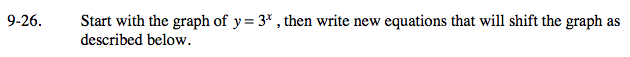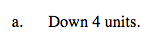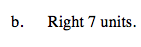### Home > A2C > Chapter 9 > Lesson 9.1.1 > Problem9-26

9-26.
1. Start with the graph of y = 3x , then write new equations that will shift the graph as described below. Homework Help ✎

1. Down 4 units.

2. Right 7 units.y = 3x − 4y = 3x − 7